## 正文

### 效果: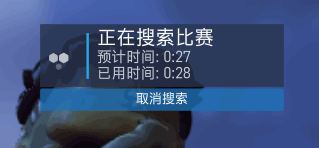### 实现过程及原理

1.绘制正六边形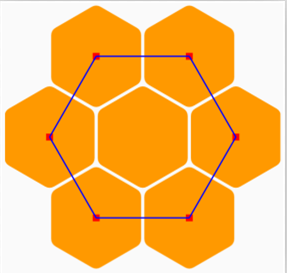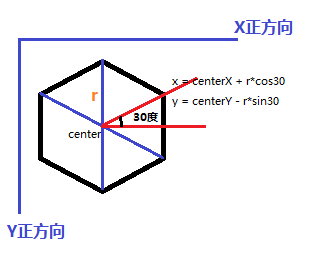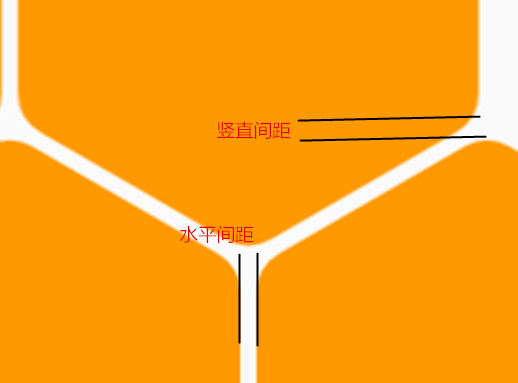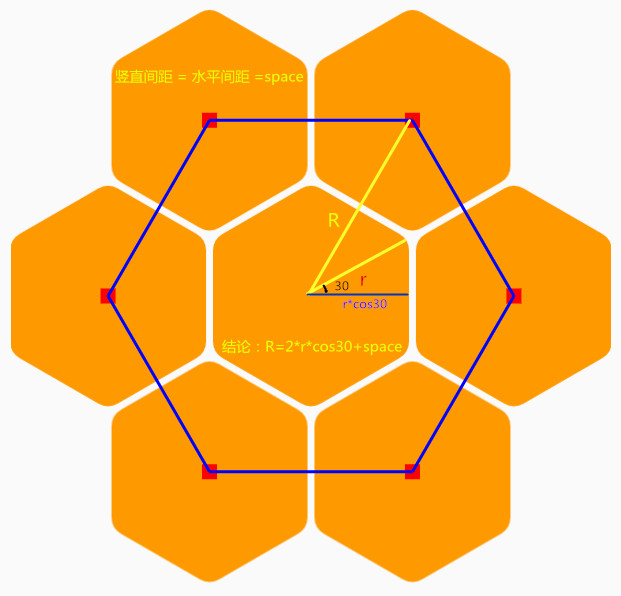@Override
protected void onMeasure(int widthMeasureSpec, int heightMeasureSpec) {
super.onMeasure(widthMeasureSpec, heightMeasureSpec);
viewHeight = getMeasuredHeight();
viewWidth = getMeasuredWidth();
if (viewWidth != 0 && viewHeight != 0) {
center.x = viewWidth / 2f;
center.y = viewHeight / 2f;
...
...
}
}

private void initHexagonCenters() {
float bigR = (float) ((1.5 * hexagonRadius + space) / cos30);
hexagonCenters = new Point(center.x - bigR * sin30, center.y - bigR * cos30);
hexagonCenters = new Point(center.x + bigR * sin30, center.y - bigR * cos30);
hexagonCenters = new Point(center.x + bigR, center.y);
hexagonCenters = new Point(center.x + bigR * sin30, center.y + bigR * cos30);
hexagonCenters = new Point(center.x - bigR * sin30, center.y + bigR * cos30);
hexagonCenters = new Point(center.x - bigR, center.y);
//左上角为第0个，中心六边形为第6个
for (int i = 0; i < 6; i++) {
}
}

private class Point {
public float x, y;

public Point(float x, float y) {
this.x = x;
this.y = y;
}

public Point() {
}
}

/**
* 六边形
*/
private class Hexagon {

//缩放值
private float scale = 1;
//透明度
private int alpha = 255;
public Point centerPoint;
//六个顶点
private Point[] vertexs = new Point;

public Hexagon(Point centerPoint, float radius) {
this.centerPoint = centerPoint;
calculatePointsPosition();
}

public void drawHexagon(Canvas canvas, Paint paint) {
paint.setAlpha(alpha);
canvas.drawPath(getPath(), paint);
}

private int calculatePointsPosition() {
if (centerPoint == null) {
return -1;
}
//从最上方顺时针数1-6给各顶点标序号 共6个点,scale作为动态参数，用以控制六边形的大小，围绕中心点做缩放变化
vertexs = new Point(centerPoint.x, centerPoint.y - radius * scale);
vertexs = new Point(centerPoint.x + radius * cos30 * scale, centerPoint.y - radius * sin30 * scale);
vertexs = new Point(centerPoint.x + radius * cos30 * scale, centerPoint.y + radius * sin30 * scale);
vertexs = new Point(centerPoint.x, centerPoint.y + radius * scale);
vertexs = new Point(centerPoint.x - radius * cos30 * scale, centerPoint.y + radius * sin30 * scale);
vertexs = new Point(centerPoint.x - radius * cos30 * scale, centerPoint.y - radius * sin30 * scale);
return 1;
}

//根据六个顶点绘制path
private Path getPath() {
Path path = new Path();
for (int i = 0; i < 6; i++) {
if (i == 0)
path.moveTo(vertexs[i].x, vertexs[i].y);
else
path.lineTo(vertexs[i].x, vertexs[i].y);
}
path.close();
return path;
}
...
...
}


2.动画实现

//更新数据的线程
private Runnable animRunnable = new Runnable() {
@Override
public void run() {
try {
while (runAnim) {
flush();
draw();
}
} catch (InterruptedException e) {
e.printStackTrace();
}
}
};

/**
* 更新数据
*/
private void flush() {
if (nowAnimatorFlag == ShowAnimatorFlag) {//逐个显示出来
for (int i = 0; i < hexagons.length - 1; i++) {
if (hexagons[i].getScale() >= scaleCritical) {
}
}

if (hexagons.getScale() == 1) {//当最后一个六边形都完全显示时，切换模式，下一轮逐个消失
nowAnimatorFlag = HideAnimatorFlag;
}

} else {//逐个消失
hexagons.subScale();
hexagons.subAlpha();
for (int i = 0; i < hexagons.length - 1; i++) {
if (hexagons[i].getScale() <= 1 - scaleCritical) {
hexagons[i + 1].subScale();
hexagons[i + 1].subAlpha();
}
}
if (hexagons.getScale() == 0) {//当最后一个六边形都完全消失时，切换模式，下一轮逐个开始显示
nowAnimatorFlag = ShowAnimatorFlag;
}
}
}

/**
* 绘制图案
*/
private void draw() {
Canvas canvas = surfaceHolder.lockCanvas();
paint.setXfermode(new PorterDuffXfermode(android.graphics.PorterDuff.Mode.CLEAR));
canvas.drawPaint(paint);
paint.setXfermode(new PorterDuffXfermode(android.graphics.PorterDuff.Mode.DST_OVER));

for (int i = 0; i < 7; i++) {
hexagons[i].drawHexagon(canvas, paint);
}
surfaceHolder.unlockCanvasAndPost(canvas);
}

## 尾声©️2019 CSDN 皮肤主题: 大白 设计师: CSDN官方博客